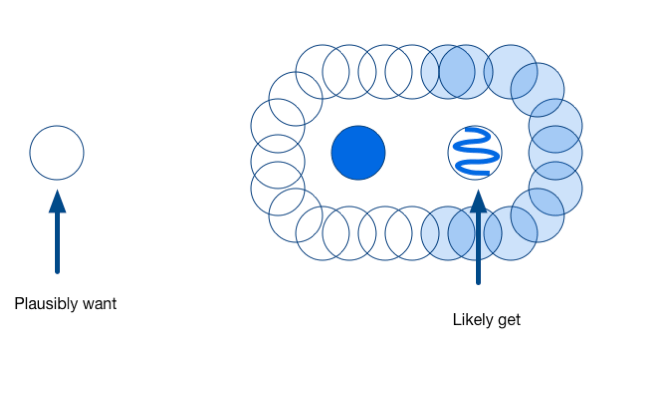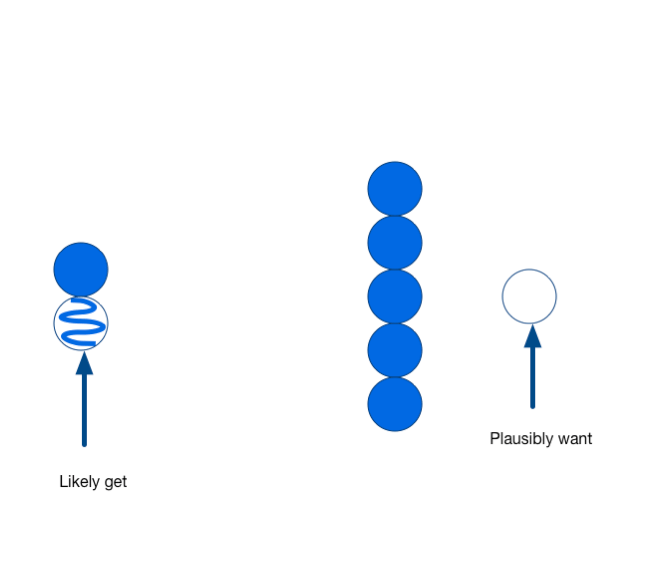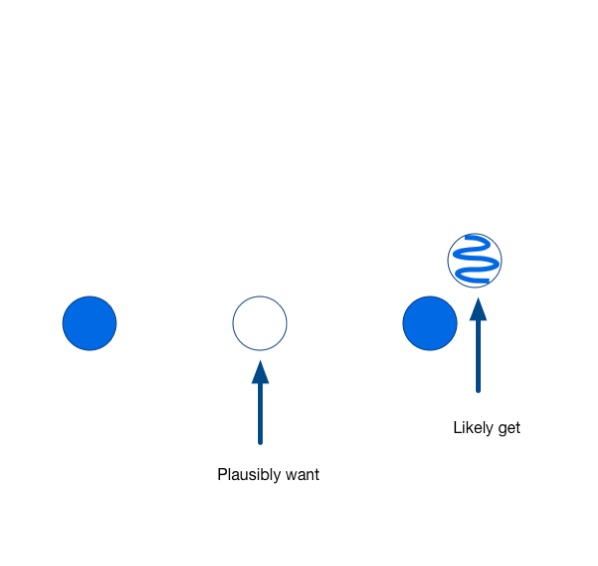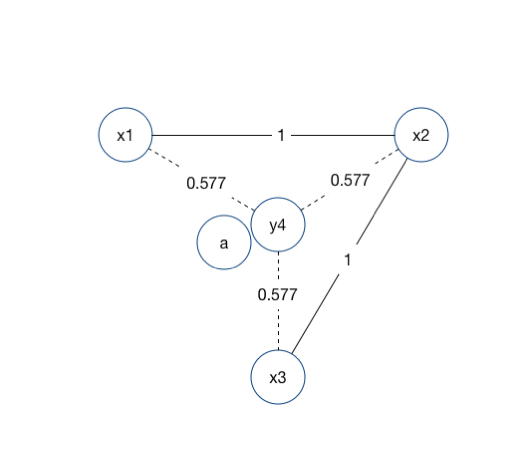# Neglected optimization topic: set diversity

The mathematical concept of set diversity is a somewhat neglected topic in current applied decision sciences and optimization. We take this opportunity to discuss the issue.

## The problem

Consider the following problem: for a number of items `U = {x_1`, … `x_n}` pick a small set of them `X = {x_i1, x_i2, ..., x_ik}` such that there is a high probability one of the `x in X` is a “success.” By success I mean some standard business outcome such as making a sale (in the sense of any of: propensity, appetency, up selling, and uplift modeling), clicking an advertisement, adding an account, finding a new medicine, or learning something useful.

This is common in:

• Search engines. The user is presented with a page consisting of “top results” with the hope that one of the results is what the user wanted.
• Online advertising. The user is presented with a number of advertisements in enticements in the hope that one of them matches user taste.
• Science. A number of molecules are simultaneously presented to biological assay hoping that at least one of them is a new drug candidate, or that the simultaneous set of measurements shows us where to experiment further.
• Sensor/guard placement. Overlapping areas of coverage don’t make up for uncovered areas.
• Machine learning method design. The random forest algorithm requires diversity among its sub-trees to work well. It tries to ensure by both per-tree variable selections and re-sampling (some of these issues discussed here).

In this note we will touch on key applications and some of the theory involved. While our group specializes in practical data science implementations, applications, and training, our researchers experience great joy when they can re-formulate a common problem using known theory/math and the reformulation is game changing (as it is in the case of set-scoring).Minimal spanning trees, the basis of one set diversity metric.

Typically a first step towards solving this problem is to build a model `z()` such that `z(x_i)` is a good estimate of the probability of a single item `x_i` “being a success.”

The usual ways to evaluate a score `z()` (precision, recall, accuracy, sensitivity, specificity, deviance, ROC/AUC) deliberately (for the sake of simplicity) ignore any intended business use or application of the model score `z()`. What we mean is using a score like `z()` to rank or sort individuals items does not necessarily solve the original problem of building a good small set of results. Good sorting may not be enough to ensure a good set.

To explain: suppose we present a small number of candidates (say 5) and consider the entire process a success if at least one of them is good. We assume our application doesn’t particularly value having multiple competing successes, as we expect successes to be mutually exclusive for any number of reasons including:

• The user might pursue only one opportunity no matter how many seem to match.
• The probabilities may be modeling a mixture of disjoint mechanisms. The user may have only one (unknown) taste, and we increase our odds of hitting it through diverse selection.

What we have to keep in mind: different sets of 5 candidate matches where each individual match individually has a 20% chance of working can have an overall chance of having any matches at all (our goal) with any probability anywhere from 100% (when the matching mechanisms are completely disjoint and complementary) to 20% (when the items are near duplicates of each other). The ability to at least attempt to bias our selection to a much higher “join value” set can make a huge operational difference.

Of course, in discussing the implications of how we are going to apply or use a model we are moving from machine learning into the field of operations research, but let us continue.

## The formal framework

We are trying to pick a small set `X` so that the probability there is an `x in X` such that `x` is a “success” is maximized. This is different than picking `X` such that the expected number of successes in `X` is maximized and different than picking `X` such that sum of `z(x)` is maximized.

The key observation is we are working against a situation of diminishing returns. For example: two perfectly good opportunities don’t double our chances when combined if they are in fact two versions of the same opportunity. The common heuristic that the value of a set is approximately the sum of the value of the elements breaks down.

The exact objective function we are interested in is:

`f(X) = P[number_successes(X)>0]`

where `X` is a set and `P[]` is probability calculated over some (usually unknown) joint probability distribution. Even if we assume our score `z(x)` is a perfect estimate of `P[x is a success]` we can’t yet estimate `P[number_successes(X)>0]` because we still would not know the probability of joint events (example: knowing `P[x1 succeeds]` and `P[x2 succeeds]` does not mean we necessarily know `P[x1 succeeds and x2 succeeds]`).

One thing `P[]` tells us is if set-success in our selected set is behaving like coin-flips (in an independent and sub-additive way), shuffled cards (in a disjoint and additive manner), or useless duplicates (in a sub-additive manner). Obviously the expected number of successes are additive, but if there is a “one or more successes” tends to be sub-additive (because two successes get the same credit as one).

We are saying the following usual additive measure:

`N(X) := sum({z(x) | x in X})`

may be convenient for the data scientist, but it is not necessarily a good stand-in for our actual application goal (such as maximizing `P[number_successes(X)>0]`), and therefore may not always pick an actual high-utility set (even when there is one).

## Working around `P[number_successes(X)>0]`

We usually do not know `P[number_successes(X)>0]`. We might get access to training data about `P[number_successes(X)>0]` (or what a computer scientist would call “a `P[number_successes(X)>0]` oracle”) by submitting sets `X` to users and recording which sets have a success (instead of submitting items and recording which items are successful). However, set based learning can introduce its own difficulties (for example see “A Note on Learning from Multiple-Instance Examples”, Avrim Blum, Adam Kalai).

What we do is: introduce a stand-in set valued function that we hope behaves somewhat like `P[number_successes(X)>0]`, allowing us to make good choices. Suppose we have models for both `z(x)` (the probability of success, or expected value of individual items `x`) and pairwise dissimilarity `d(x1,x2)` (presume `d(,)` is designed to be in the range zero to one, with zero indicating perfect similarity).

One might hope that with access to `z()` and `d(,)` one could appeal to something like inclusion/exclusion and define a useful stand-in function that is a sum of the `z()`s plus all the pair `d(,)`s. Because there are so many more `d(,)`s than `z()`s such function does not always point us to reasonable set selections. Later on we show a principled method to control the number of `d(,)`s and get a very useful stand in function.

More commonly researchers use covering or variance heuristics as stand-ins the unknown set-valued function `f(X) = P[number_successes(X)>0]`.

### Coverage measure

A good stand-in function is a coverage measure such as `Z()`:

`Z(X) := sum({z(v) | exists x in X and v in V such that d(x,v) ≤ a})` where `V` is a “universe” of items we are trying to simultaneously be near to or cover. We can take `V=U` (the set we are choosing from), or (better, but introduces a circular appeal to sovling the coverage/diversity/discrepancy problem) `V` as a low discrepancy sample with respect to the unknown set-distribution of successes `P[]`.

The idea is: if we cover all good opportunities we should have good chance having a hit. This has been used in literature for biological sampling, Adwords sorting (for example see: “Revisiting the greedy approach to submodular set function maximization”, PR Goundan, AS Schulz – Optimization online, 2007), and other applications.

Coverage style measures yield rich combinatorial optimization problems, especially when you value sets of items in terms of what composite systems can be assembled from them (such as drink recipes from ingredients, as cleverly illustrated by Jordan Meyer).

### Total variation measure

One desire is for the measure to be intrinsic, or only depend on the items selected (and be immune to changes in the set of items that can be selected from, the idea is the distribution of where we can look may be a misleading estimate of where we want to look).

A common intrinsic stand-in function is the variation measure `Z'()`:

`Z'(X) := sum({d(x,y) | x,y in X; z(x) ≥ a, z(y) ≥ a})`.

This one has been used in molecular diversity, and is what you would get from an inclusion/exclusion style argument.

### Geometric measures

In theory one could try a number of geometrically inspired measures based on the following (though most are impractical to actually implement).

Pick `d`, a dimension to work in and for a set of items `X` let `X'` be `{ x in X | z(x) ≥ a }`. Use distance geometry methods to define a function `g()` from `X'` to `R^d` such that `||g(x)-g(y)|| ~ d(x,y)` for all `x,y in X'`. With such a coordinatization in hand we can use various geometric quantities as diversity measures:

• Determinant of the inertial ellipsoid of the `g(x)`.
• Volume of the convex hull of the `g(x)`.
• Total volume of the union of unit-radius spheres centered at each `g(x)`.

## Submodularity

One should prefer a function like `Z()` over many of the others because `Z()` is efficiently implementable and captures the idea of diminishing returns: each point added tends to have less of an improvement than the previous. The unknown true objective `f(X) = P[number_successes(X)>0]` has the diminishing return property, so we would expect a good stand-in function to also have such a property. Many of the other common measure (such as total variation) lack this feature. The theory of submodular functions was invented to study optimization over diminishing returns and is a key concept in the literature (see also: “Submodular Function Maximization”, Andreas Krause (ETH Zurich), Daniel Golovin (Google)).

Let’s look at set-valued stand in functions `M()` that are:

• Monotone. That is `M(Y) ≥ M(X)` when `X` is contained in `Y`.
• Submodular. That is `M(X) + M(Y) ≥ M(X union Y) + M(X intersect Y).`

This may seem a bit abstract. But it is designed to model situations of diminishing returns. The following common functions are all provably monotone and submodular:

• Set cardinality.
• Weighted set cover (`Z()`, and other functions often used in the literature).
• Volumes of unions of objects.
• `P[number_successes(X)>0]` the (unknown) probability of a set containing a successful item.

The ideas are:

• Maybe all of these functions behave similarly (so optimizing one informs us about the optimum of another).
• While fully maximizing a non-negative monotone submodular function is often intractable, it is a theorem that the so-called “greedy algorithm” achieves a maximum that is at least `(1-1/e)` of the optimum value. Only getting within 63% of the optimum utility may seem like giving up a lot, but it is a lot better than only being within 20% of the optimal utility (as in our earlier 5 element example). In fact if success methods are disjoint (which is plausible) and one class is over-represented (also plausible) we would with very high probability see 20% utility for an item oriented selection. In this situation the greedy set coverage selection is likely over three times as effective as the naive selection (as `(1-1/e)/.2 > 3`)! For the math: Nemhauser, George L., Laurence A. Wolsey, and Marshall L. Fisher. “An analysis of approximations for maximizing submodular set functions—I.” Mathematical Programming 14.1 (1978): 265-294.

## A non-submodular heuristic

In 1999 I published an additional heuristic set value stand-in function that I had been using and publicly speaking on for some years. It was inspired by clustering algorithms and the desire to control which terms enter into an inclusion/exclusion style argument (via something like a dependency tree).

This function is unfortunately not monotone or submodular even with Euclidian distances. However, it has a number of desirable properties complementary to common coverage or variance measures (see: “IcePick:  A Flexible Surface-Based System for Molecular Diversity”, John Mount, Jim Ruppert, Will Welch, and Ajay N. Jain, J. Med. Chem., 1999, 42 (1), pp 60–66.). This stand-in function works very well, and could be profitably applied in many applications.

Abstractly the function is defined as:

`S(X)` is defined as the total length of edges in
the minimum weight spanning tree formed on the graph of nodes `{G | x in X such that z(x) ≥ a}` with edge-weights given by `d(,)`.

An obvious variation of `S()` is to re-process the `d(,)` into something like `d'(x,y) = (z(x) + z(y)) d(x,y)/2` to lean a bit more towards individual values. We can also add `max_x z(z)` to `S()` so that `S()` has a meaningful value on single item sets.

I designed this stand-in diversity function `S()` to have the following properties:

• Inexpensive to compute.
• Valuing distance (or aperture), items/points further away tend to be worth more as they are more extreme measurements (not a property of coverage measures such as `f(X) = P[number_successes(X)>0]`).
• Intrinsic. `S(X)` should be a function of only `X` (the set chosen to score), and not of a larger ambient cover target set `U` (where we are choosing from). This was critical in our application as `U` was varying as chemists proposed more molecules.
• No credit for presumed bad items (those that have `z(x) < a`). We note that `f(X) = P[number_successes(X)>0]` itself is not an intrinsic score, but we are assuming that the distribution of available items `U` is likely a very misleading estimate of the measure `P[]` we are trying to cover.
• No credit for presumed duplicates, `S(X) = S(X union {y})` when there is a `x in X` such that `d(x,y)=0`.
• Consistent across selection sizes. Roughly: `S(X)` should be linear in `|X|` for good diverse targets (that is `X` where `z(x) ≥ a` and `d(x,y)=1` for all `x,y in X`. This allows us to use the scores to help pick selection size.
• Near additivity. Adding a new good item that is at least distance `d` from all other items should add about `d` units of score. This is not a feature shared by the probability problem we motivated this note with (or by `Z()` or `Z'()`. This is because `S()` was designed for scientific experiment design where extreme examples were thought to be more valuable in learning underlying mechanism. So we were not hoping for a biological “hit” on the first screen, but instead a screen that returned a lot of information due to having a lot of useful variation.
• Diminishing returns (though not in the exact same sense as submodular functions): `S(X union Y) ≤ S(X) + S(Y) + min_{x in X, y in Y} d(x,y)`.

Total variation measures were in use at the time, and it was widely thought that in this field coverage style measures would be overwhelmed by the streetlight effect (the distribution of what we could most easily inspect being a very bad, even misleading, stand-in for the distribution induced by `P[]`) especially optimizing over molecular libraries produced by combinatorial chemistry methods (once derided as “looking for a needle in a haystack by building more haystacks.”).“Mutt and Jeff” 1942, the streetlight effect (looking in popular/easy spots instead of high-value spots).

To find a good set I used a 2-opt optimization heuristic over the score `S()`. This dominates the greedy algorithm as long as you use a greedy solution as one of your optimization attempt starts (though we did not prove an approximation bound on optimality in this case as the greedy/submodular argument does not immediately apply in this case).

I feel the spanning tree measure is complementary to commonly used coverage and variance set measures and serves well in many set-valuation projects.

## Choosing a heuristic

It is our conjecture that there may not be one efficient intrinsic diversity or discrepancy measure “to rule them all”. Your choice of diversity measure should depend on your problem domain and how good an estimate you have of the unknown joint distribution you can acquire. It is likely something like Arrow’s impossibility theorem applies and there may be no measure simultaneously modeling all reasonable demands.

Instead we suggest trying a few examples (both notional and real) and seeing which heuristic tends to steer towards expected “right” answers. Each of the three main efficiently implementable measures (coverage, variation, and spanning measure) have a digram they clearly do poorly on. But keep in mind: even a reasonable attempt to score set value as a sub-additive function can greatly outperform simply adding individual item scores.

We illustrate a instruction situations below. In each case we have a number of items we can select (the circles), some of which are already selected (the filled circles). Distances are as viewed in the diagram. The problem is to choose the next item to select (perhaps part of a greedy allocation). In each case we show an plausible intuitive good pick, and show the contrary item the measure will likely pick (a bad choice avoided by one of the competing measures).Example 1: coverage measure distracted from useful diversity by over-coverage of one region. This was the motivating problem in our original biotech application as combinatorial chemistry can produce huge numbers of related molecules- independent of the value of exploring a particular region of biological behavior space.Example 2: variance measure distracted from usefully exploring new space by exploiting simultaneous distance to many already selected items. We saw this flaw in actual applications: wastefully picking essentially duplicate molecules for experiment because it appeared to represent more chances to be far away from another biological motif.Example 3: spanning tree measure distracted from useful subdivision by collinearity. Spanning tree measure over-value the boundary. The problem is greatest if your search space is “small” (or elliptic) and less if your search space is “big” (or hyperbolic).

Overall the coverage measure is the most natural. The main issue with it is the distribution of available items may very much mis-represent the (unknown) measure or density induced by the true valuation density. We used the spanning tree measure because this mismatch of density (in the presence of molecular libraries produced by combinatorial chemistry methods) was considered the plausibly biggest issue in our original biotech application.

## Conclusion

For set oriented applications (such as showing a user a page of search results) you must move from individual item scoring or ranking to set-valued scoring. Set valued optimization can be counterintuitive, complicated and expensive- but can make a massive difference in the quality of your final application.

## Why the spanning tree measure is not monotone or submodular

The reason the spanning tree measure is not monotone or submodular (even for Euclidian dissimilarity measures) is given by Steiner style examples such as the following:In the above diagram define the sets: `X = {}` and `Y = {y1, y2, y3}`. We see the minimal spanning tree on `(Y union {a})` is shorter than the minimal spanning tree on `Y` (violating monontonicity).

For our next example consider `X = {x1, x2, x3}` and `Y = {x1, x2, x3, y4}`. Then we have `X contained in Y` and as “Y has already sprung the trap” we have `S(X union {a}) - S(X)` is negative and `S(Y union {a}) - S(Y)` violating submodularity (which would require `S(X union {a}) - S(X) ≥ S(Y union {a}) - S(Y)`).(edit): Just wanted to call out an excellent article on the topic of greedy algorithms and approximately maximizing submodular functions: When Greedy Algorithms are Good Enough: Submodularity and the (1 – 1/e)-Approximation.### jmount

Data Scientist and trainer at Win Vector LLC. One of the authors of Practical Data Science with R.

### 2 replies ›

1.Greg says:

Has work been done to address the case where U actually does affect P(number successes > 0)?

That seems analogous to the case of a recommender system serving suggestions to a consumer, in which case the probability of them buying one of the recommended items depends on the overall item selection.

1.John Mount says:

Great question. I am sure somebody has looked at this in retail pricing (I think relevant terms are “decoy effect” and “menu dependance” plus “The Paradox of Choice.”). Jordan Meyer’s cocktail ingredient scoring problem (I mentioned this briefly in the article) also has similar structure.

But I don’t have a good answer. I am not familiar with the literature in this direction and what the standard structural assumptions for problems of that nature are (as such set-valued f() can be pretty wild).